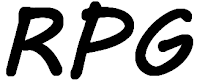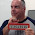## Wednesday, April 9, 2014

### Add 1 to A to get BMy colleagues and I came up with an interesting problem earlier: I have a 3 long field and I want to increment the value in it. We need to have the maximum possible number of values in the field before it returns to the initial value.

If I use numbers I have 1,000 values, 0 – 999.

If I used alphanumeric values for a range of 000 – ZZZ I would have 46,656 values. But how can I add 1 to the letter A?

This scenario is on one of our IBM i server partitions. We do not have the programming language C++ or Java, just CL and RPGLE.

If you define a variable in RPG as alphanumeric it is not possible to add 1 to it, see below:

 ``` dcl-s TestValue char(3) inz('000') ; TestValue += 1 ; C ADD 1 TESTVALUE *inlr = *on ; COMPILE ERRORS Msg id Sv Statmnt Message text RNF7421 30 000300 Operands are not compatible with the type of operator. RNF7421 30 000300 Operands are not compatible with the type of operator. RNF7044 30 000400 The field TESTVALUE on the C specification is not numeric; the specification is ignored.```

After much debate I came up with the following method using two constants and the %XLATE built in function.

In this example the value will be written to a file, TESTPF, which define as:

 ``` A R TESTPFR A TESTVALUE 3A```

In the new RPG "all free” this file is coded as:

 `01 dcl-f TESTPF usage(*output) ;`

I also need two constant, which are defined with dcl-c, and a "counter” field which is defined using dcl-s:

 ```02 dcl-c Before '0123456789ABCDEFGHIJKLMNOPQRSTUVWXYZ' ; 03 dcl-c After '123456789ABCDEFGHIJKLMNOPQRSTUVWXYZ0' ; 04 dcl-s i uns(5) ;```

Notice that the two constants are, I cannot think of a better word, "staggered". Before starts with zero, and After with 1.

I use a FOR operation to perform 10,000 increments of TESTVALUE, see below:

 ```05 TestValue = '000' ; 06 for i by 1 to 10000 ; 07 if (%subst(TestValue:3:1) = 'Z') ; 08 exsr Increment ; 09 else ; 10 %subst(TestValue:3:1) 11 = %xlate(Before:After:%subst(TestValue:3:1)) ; 12 endif ; 13 write TESTPFR ; 14 endfor ;```

First I have to test to see if I need to "roll over” from a Z to a zero in the third position, line 7. If I do I will perform the subroutine Increment, line 8, which I will explain below.

If I do not have to "roll over” I perform the %XLATE built in function. As the values in the constants are "staggered" zero is translated to 1, 1 to 2, etc. until I come to 9. 9 is translated to A, A to B, etc. until I reach to Z. This translation sort of emulates adding 1 to a character whether or not it is a number. I cannot translate Z to zero without incrementing the second position, hence the statements on lines 7 and 8.

The subroutine Increment starts by "rolling over" the third position to zero, line 17 below.

 ```16 begsr Increment ; 17 %subst(TestValue:3:1) = '0' ; 18 if (%subst(TestValue:2:1) = 'Z') ; 19 %subst(TestValue:2:1) = '0' ; 20 if (%subst(TestValue:1:1) = 'Z') ; 21 %subst(TestValue:1:1) = '0' ; 22 else ; 23 %subst(TestValue:1:1) = %xlate(Before:After:%subst(TestValue:1:1)) ; 24 endif ; 25 else ; 26 %subst(TestValue:2:1) = %xlate(Before:After:%subst(TestValue:2:1)) ; 27 endif ; 28 endsr ;```

I think the code above is pretty self evident.

The second position is "incremented", line 26, if it does not need to be "rolled over" to zero, line 21, as it is equal to Z. If it was "rolled" then the first position either needs to be incremented, line 23, or "rolled”, line 21.

If you know of a better way please send it to me via the Contact Form on the right, rather than post it as a comment. That way I can make it into a post and give you credit for it.

You can learn more about the %XLATE built in function in RPGLE on the IBM website here.

1.I think you can replace lines 7 through 12 with the following and not need subroutine INCREMENT.

%subst(TestValue:3:1) = %xlate(Before:After:%subst(TestValue:3:1)) ;
if (%subst(TestValue:3:1) = '0';
%subst(TestValue:2:1) = %xlate(Before:After:%subst(TestValue:2:1)) ;
if (%subst(TestValue:2:1) = '0';
%subst(TestValue:1:1) = %xlate(Before:After:%subst(TestValue:1:1))
endif;
endif;

I did not test this but I believe it works.

2.I am thinking there must be a better mathematical way to translate. Sort of like translating between hex and decimal. When you convert hex 'FF' to decimal you don't use arrays or string processing. Decimal is base 10 numbering, Hex is base 16. This could be a base 36 numbering scheme. And each digit starting from the right side is increased by 36 power. For a 3 digit number 1XA you take A * 36^0 + X * 36^1 + 1 * 36^2.
A = 11, X = 33, so (11 * 1) + (33 * 36) + (1 * 1296) = 2495
But you would still need to convert the base 36 character to a number using an array most likely. I did find some interesting info on it including some translation code here: http://en.wikipedia.org/wiki/Base_36. One thing you might want to consider is using Hex instead and then you can use some existing translation methods.
Doug Bridwell

3.I tested this on my very old V5R2 iSeries. It has been sitting in my basement for quite some time and I was looking for an excuse to power it on. I wonder which version of code runs faster?

D AlphaIncrement...
D PR
D byte3 3a
D*-----------------------------------------------------
D AlphaNumber...
D s 3a inz('000')
D count...
D s 10u 0
D*-----------------------------------------------------
/Free
*inlr = *on;
for count = 1 to 50000;
AlphaIncrement(AlphaNumber);
dsply AlphaNumber;
endfor;
return;
/End-Free
P AlphaIncrement...
P B
D AlphaIncrement...
D PI
D byte3 3a
D*-----------------------------------------------------
D l_DS...
D DS
D l_AlphaNum...
D 1 3a
D l_int100...
D 1 1u 0
D l_int10...
D 2 2u 0
D l_int1...
D 3 3u 0
/Free
l_AlphaNum = byte3;
l_int1 += 1;

select;
when (l_int1 = X'FA');
l_int1 = X'C1';
when (l_int1 = X'CA');
l_int1 = X'D1';
when (l_int1 = X'DA');
l_int1 = X'E2';
when (l_int1 = X'EA');
l_int1 = X'F0';
l_int10 += 1; endsl;

select;
when (l_int10 = X'FA');
l_int10 = X'C1';
when (l_int10 = X'CA');
l_int10 = X'D1';
when (l_int10 = X'DA');
l_int10 = X'E2';
when (l_int10 = X'EA');
l_int10 = X'F0';
l_int100 += 1;
endsl;

select;
when (l_int100 = X'FA');
l_int100 = X'C1';
when (l_int100 = X'CA');
l_int100 = X'D1';
when (l_int100 = X'DA');
l_int100 = X'E2';
when (l_int100 = X'EA');
l_int1 = X'F0';
l_int10 = X'F0';
l_int100 = X'F0';
endsl;

Byte3 = l_AlphaNum;
/End-Free
P AlphaIncrement...
P E

4.Couldn't resist offering an alternative. To be truly effective mine relies on keeping the "number" as a array of 1 byte integers and then having the increment routine return the character version. In production code I'd separate the increment and the translate to character functions because I hate functions that modify the input as this does. Anyway it was a fun little exercise. I suspect mine is more efficient - it runs very quickly - and it adapts very nicely if you wanted to change it to a 4, 5, 6, .... "digit" number. I'm sure I could shorten it, but it's bed time.

Thanks for the challenge - that was fun.

dcl-s charNumber char(3);

dcl-ds realNumber;
digit int(3) Dim(3) Inz;
End-Ds;

dcl-s x int(5);

for x = 1 to 2000;
charNumber = increment(realNumber);
if %Rem( x: 360 ) = 0;
dsply ('At ' + %char(x) + ' number is "' + charNumber + '"');
EndIf;
EndFor;

*InLR = *On;

dcl-proc increment;

dcl-pi increment char(3);
inputNumber likeDs(realNumber);
end-pi;

dcl-s i int(5);
dcl-s charWork varchar(3);
dcl-s overflow ind;

dcl-ds chars;
*n char(36) Inz('0123456789ABCDEFGHIJKLMNOPQRSTUVWXYZ');
character char(1) pos(1) dim(46);
end-ds;

charWork = ''; // Set workfield to null

For i = %elem(inputNumber.digit) downto 1;
overflow = *Off;
inputNumber.digit(i) += 1;
If inputNumber.digit(i) > 35;
inputNumber.digit(i) = 0;
overflow = *On;
EndIf;
If overflow = *Off;
Leave;
Else;
If i = 1; // Already on high digit so overflow is an error
Dsply 'Overflow error - "number" too large';
EndIf;
EndIf;
EndFor;

For i = 1 to %elem(digit);
charWork += character( inputNumber.digit(i) + 1 );
EndFor;

Return charWork; // All done

end-proc;

5.After I posted my first solution I came up with a much better, shorter, version. Following SImon's instructions I sent it as a comment instead but it hasn't shown up yet ... hmmmm.

6.I posted a set of base 36 conversion routines and they haven't shown up either.

1.You can see your code in the post here.

7.You could use the C run-time library, which is always at your disposal. You could code something like:

n = strtol(field, *NULL, 36);
n = n + 1;
ltoa(n, field, 36);

(I'll leave the function prototypes and edge conditions to you.)

1.I would used the unsigned versions for this.

8.In "old" RPG you could use the BITON/BITOFF OP code to do that

9.You have to set up a array of characters in the order of use. Then write a function to get the net character for the set.

I have seem this mostly used with characters in the highest order position.

A1234567890 for instance. This gives you a unique key for an exponent of 10 to the 26th. Using character is every position can be done but would require more effort to get the sequence right.

In the past I have broken used this for something like an invoice number using two fields. An single Alpha character, then a Numeric of some length. When the numeric value reaches it's highest value then increment the character to the next character in order.

10.Since I like arrays so much, I would load the values in an array.
The value "0" to "Z" would be in a compile time array of course.
You can than use numeric values, but when it's time to write the final value to file you select the value from the array so it is immediately available.
Loading the array doesn't even take 0,5 seconds, but after that I never have to go through a for...next loop again.

To prevent "comment spam" all comments are moderated.
```